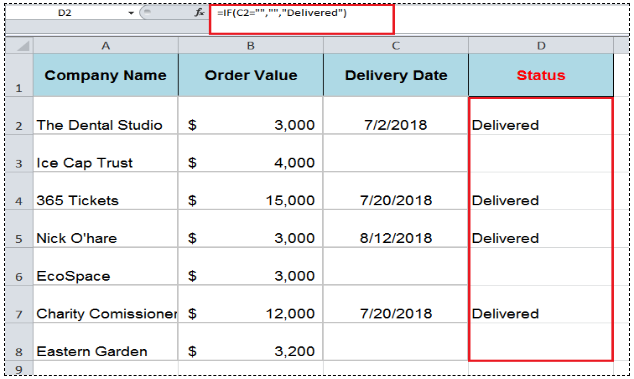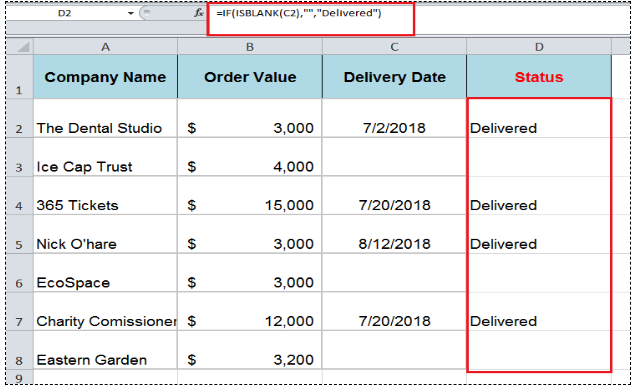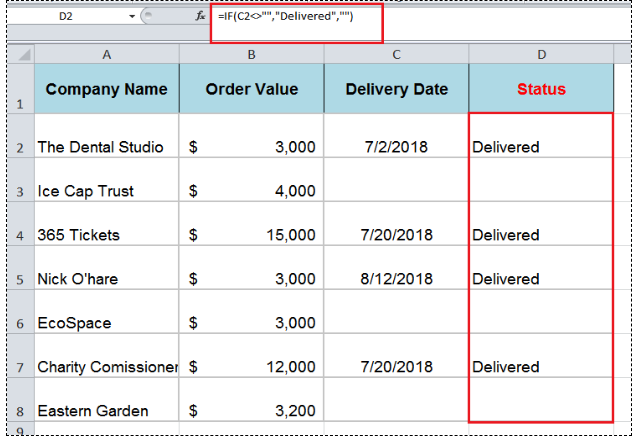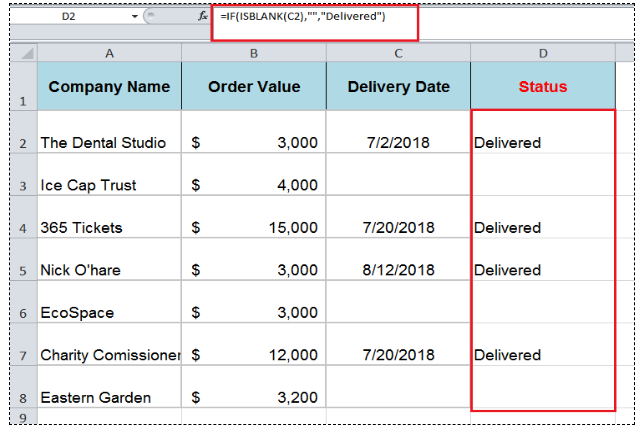Get instant live expert help with Excel or Google Sheets“My Excelchat expert helped me in less than 20 minutes, saving me what would have been 5 hours of work!”

#### Post your problem and you’ll get expert help in seconds.

Your message must be at least 40 characters
Our professional experts are available now. Your privacy is guaranteed.

# How to Determine IF a Cell is Blank or Not Blank in Excel

You may have a range of data in Excel and need to determine whether or not a cell is Blank. This article explains how to accomplish this using the IF function.

## Determine if a cell is blank or not blank

Generally, the Excel IF function evaluates where a cell is Blank or Not Blank to return a specified value in TRUE or FALSE arguments. Moreover, IF function also tests blank or not blank cells to control unexpected results while making comparisons in a logical_test argument or making calculations in TRUE/FALSE arguments because Excel interprets blank cell as zero, and not as an empty or blank cell.

### Syntax of IF function is;

`IF(logical_test, value_if_true, value_if_false)`

In IF statement to evaluate whether the cell is Blank or Not Blank, you can use either of the following approaches;

• Logical expressions Equal to Blank (=””) or Not Equal to Blank (<>””)

• ISBLANK function to check blank or null values. If a cell is blank, then it returns TRUE, else returns FALSE.

Following examples will explain the difference to evaluate Blank or Not Blank cells using IF statement.

### Blank Cells

To evaluate the cells as Blank, you need to use either logical expression Equal to Blank (=””) of `ISBLANK `function inthe` logical_test` argument of the IF formula. In both methods logical_test argument returns TRUE if a cell is Blank, otherwise, it returns FALSE if the cell is Not Blank

For example, you need to evaluate that if a cell is `Blank,` the blank value, otherwise return a value “Delivered”. In both approaches, following would be the IF formula;

`=IF(C2="","","Delivered")`

OR

`=IF(ISBLANK(C2),"","Delivered")`

In both of the approaches, `logical_test` argument returns TRUE if a cell is Blank, and the `value_if_true` argument returns the blank value. Otherwise, the `value_if_false`argument returns value “Delivered”.### Not Blank Cells

To evaluate the cells are `Not Blank` you need to use either the logical expression Not Equal to Blank (<>””) of `ISBLANK `function in` logical_test` argument of IF formula. In case of logical expression Not Equal to Blank (<>””) logical_test argument returns TRUE if the cell is Not Blank, otherwise, it returns FALSE. In case of the `ISBLANK` function, the `logical_test` argument returns FALSE if a cell is Not Bank. Otherwise it returns TRUE if a cell is blank.

For example, you need to evaluate that if a cell is `Not Blank,` then return a value `Delivered`, otherwise return a blank value. In both approaches, following would be the IF formula;

`=IF(C2<>"","Delivered","")`

In this approach, the logical expression Not Equal to Blank `(<>`“”`)` returns TRUE in the`logical_test` argument if a cell is Not Blank, and the `value_if_true` argument returns a value “Delivered”, otherwise `value_if_false` argument a blank value.OR

`=IF(ISBLANK(C2),"","Delivered")`

This approach is opposite to first one above. In IF formula, ISBLANK function returns FALSE in the`logical_test` argument if a cell is Not Blank, so `value_if_true` argument returns blank value and a `value_if_false` argument returns a value “Delivered”.Still need some help with Excel formatting or have other questions about Excel? Connect with a live Excel expert here for some 1 on 1 help. Your first session is always free.

Are you still looking for help with the IF function? View our comprehensive round-up of IF function tutorials here.

### Did this post not answer your question? Get a solution from connecting with the expert.Another blog reader asked this question today on Excelchat:
Solution examplesI have a cell that has a working vlook formula in it. When it looks up source data it returns blank. That is fine. I now want to use an IF formula to say if that cell is blank leave the other cell blank and if not then show value in different cell. The issue I have is that when the IF formula looks up the cell it reports back FALSE due to the vlook up formula being in the cell. I want the IF formula to show nothing instead of FALSE. Can you help?
Solved by Z. E. in 60 minsI have a list or people who are ranked in numbers from 3 to 6 I need to recognize the contents of each multiple cells and create an equivalent letter. for that value into another column. e.g. Cell E1 = 3 to show in new cell that row (H1) the letter "C" I have multiple rows with different values in column "E" Can this be done?
Solved by I. J. in 30 minsCould you help me by explaining the IF function?
Solved by G. L. in 24 minsI want to display a range of cells if they meet a specific criteria. I want to display a name IF it does not equal one of three options AND there are any numbers contained in a range of cells
Solved by G. F. in 60 minsI have a question about Conditional Formatting. =IF('Sheet1'!A1="X",IF('Sheet1'!B1="Y", TRUE, FALSE),FALSE) the set the cell background to red. I would like to make it so that if Sheet1'!B1="Z" I set the back to blue. How can I achieve this.
Solved by B. F. in 39 mins## Subscribe to Excelchat.coAnother blog reader asked this question today on Excelchat: